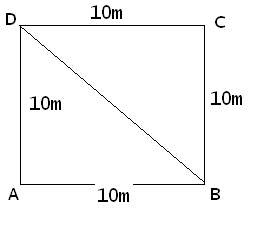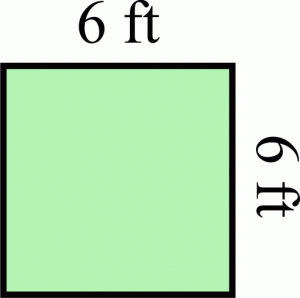## How to Calculate and Solve for the Perimeter and Length of a Square | The Calculator EncyclopediaThe image above represents a square.
To compute the perimeter of a square requires one essential parameter which is the length of one side of a square.

The formula for computing the perimeter of a square is:

P = 4 x l

Where:
P = Perimeter of a Square
l = Length of one side of a Square

Let’s solve an example
Find the perimeter of a square which has a length of 10 m.

This implies that:
l = length of one side of a square = 10

P = 4 x l
P = 4 x 10
P = 40

Therefore, the perimeter of the square is 40 m.

Calculating the Length of a Square when Perimeter is Given

The formula is l = p / 4

Where;
P = Perimeter of a square
l = Length of a square

Let’s solve an example:
Find the length of a square where the perimeter of a square is 100 m.

This implies that;
P = Perimeter of a square = 100 m

l = p / 4
l = 100 / 4
l = 25

Therefore, the length of the square is 25 m.

## How to Calculate and Solve for the Area and Length of a Square | The Calculator EncyclopediaThe image above represents a square.
To compute the area of a square requires one essential parameter which is the length of one side of a square.

The formula for computing the area of a square is:

A = l2

Where:
A = Area of a Square
l = Length of one side of a Square

Let’s solve an example
Find the area of a square which has a length of 6 ft.

This implies that:
l = length of one side of a square = 6

A = l2
A = 62
A = 6 x 6
A = 36

Therefore, the area of the square is 36 ft2.

Calculating the Length of a Square when Area is Given

The formula is l = √[A]

Where;
A = Area of a square
l = Length of a square

Let’s solve an example:
Find the length of a square where the area of a square is 81 ft2.

This implies that;
A = Area of a square = 81 ft2

l = √[A]
l = √
l = 9

Therefore, the length of the square is 9 ft.